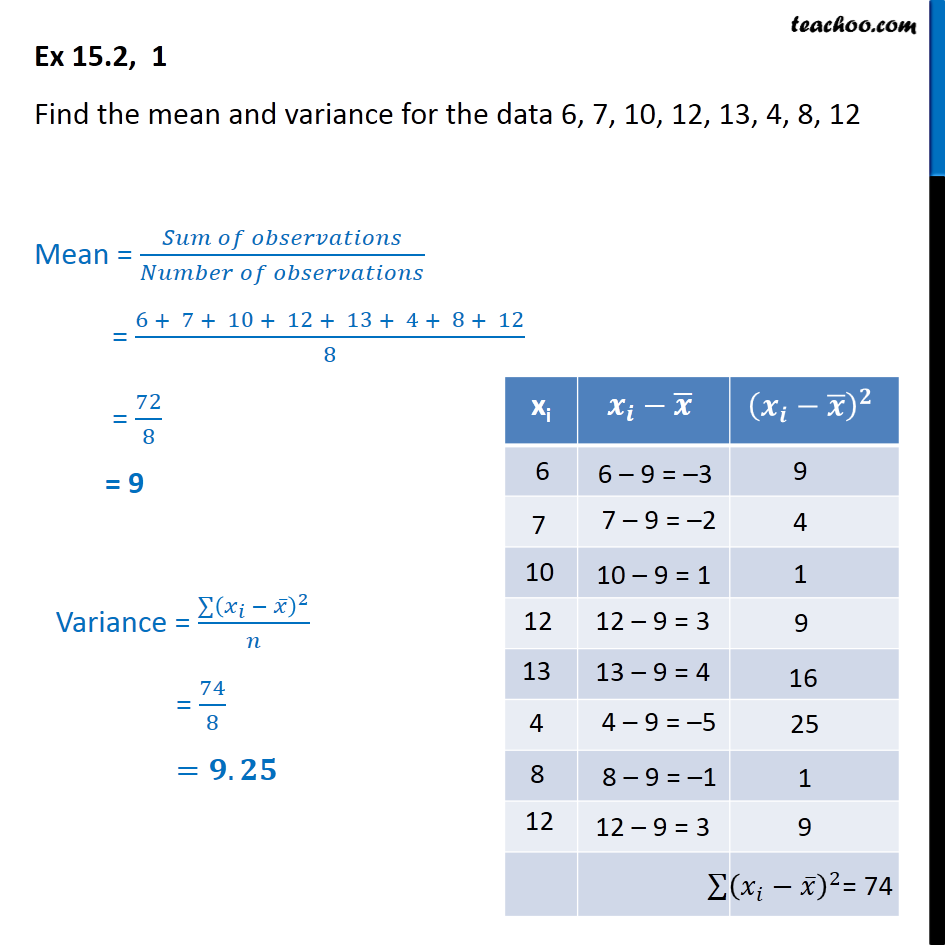Ex 13.2

Chapter 13 Class 11 Statistics
Serial order wiseLearn in your speed, with individual attention - Teachoo Maths 1-on-1 Class

### Transcript

Ex 13.2, 1 - Chapter 15 Class 11 Statistics - NCERT Find the mean and variance for the data 6 , 7, 10, 12, 13, 4, 8, 12 xi x_i-x ̅ (x_i-x ̅ )^2 6 7 10 12 13 4 8 12 6 – 9 = –3 7 – 9 = –2 10 – 9 = 1 12 – 9 = 3 13 – 9 = 4 4 – 9 = –5 8 – 9 = –1 12 – 9 = 3 9 4 1 9 16 25 1 9 ∑▒(𝑥_𝑖−𝑥 ̅ )^2 = 74 Mean = (𝑆𝑢𝑚 𝑜𝑓 𝑜𝑏𝑠𝑒𝑟𝑣𝑎𝑡𝑖𝑜𝑛𝑠)/(𝑁𝑢𝑚𝑏𝑒𝑟 𝑜𝑓 𝑜𝑏𝑠𝑒𝑟𝑣𝑎𝑡𝑖𝑜𝑛𝑠) = (6 + 7 + 10 + 12 + 13 + 4 + 8 + 12)/8 = 72/8 = 9 Variance = (∑▒(𝑥_𝑖 − 𝑥 ̅ )^2 )/𝑛 = 74/8 =𝟗.𝟐𝟓